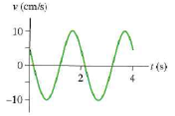# Problem: For the graph below, determine the frequency f and the oscillation amplitude A

###### FREE Expert Solution

Frequency and periodic time are related as

$\overline{){\mathbf{f}}{\mathbf{=}}\frac{\mathbf{1}}{\mathbf{T}}}$

1 Hz = 1 s-1

Angular frequency,

$\overline{){\mathbf{\omega }}{\mathbf{=}}{\mathbf{2}}{\mathbf{\pi }}{\mathbf{f}}}$

Velocity amplitude is given by:

$\overline{){{\mathbf{v}}}_{\mathbf{m}\mathbf{a}\mathbf{x}}{\mathbf{=}}{\mathbf{A}}{\mathbf{\omega }}}$

Periodic time is the time period between two successive crests.

Periodic time, T = 2 s

82% (98 ratings)###### Problem Details

For the graph below, determine the frequency f and the oscillation amplitude A